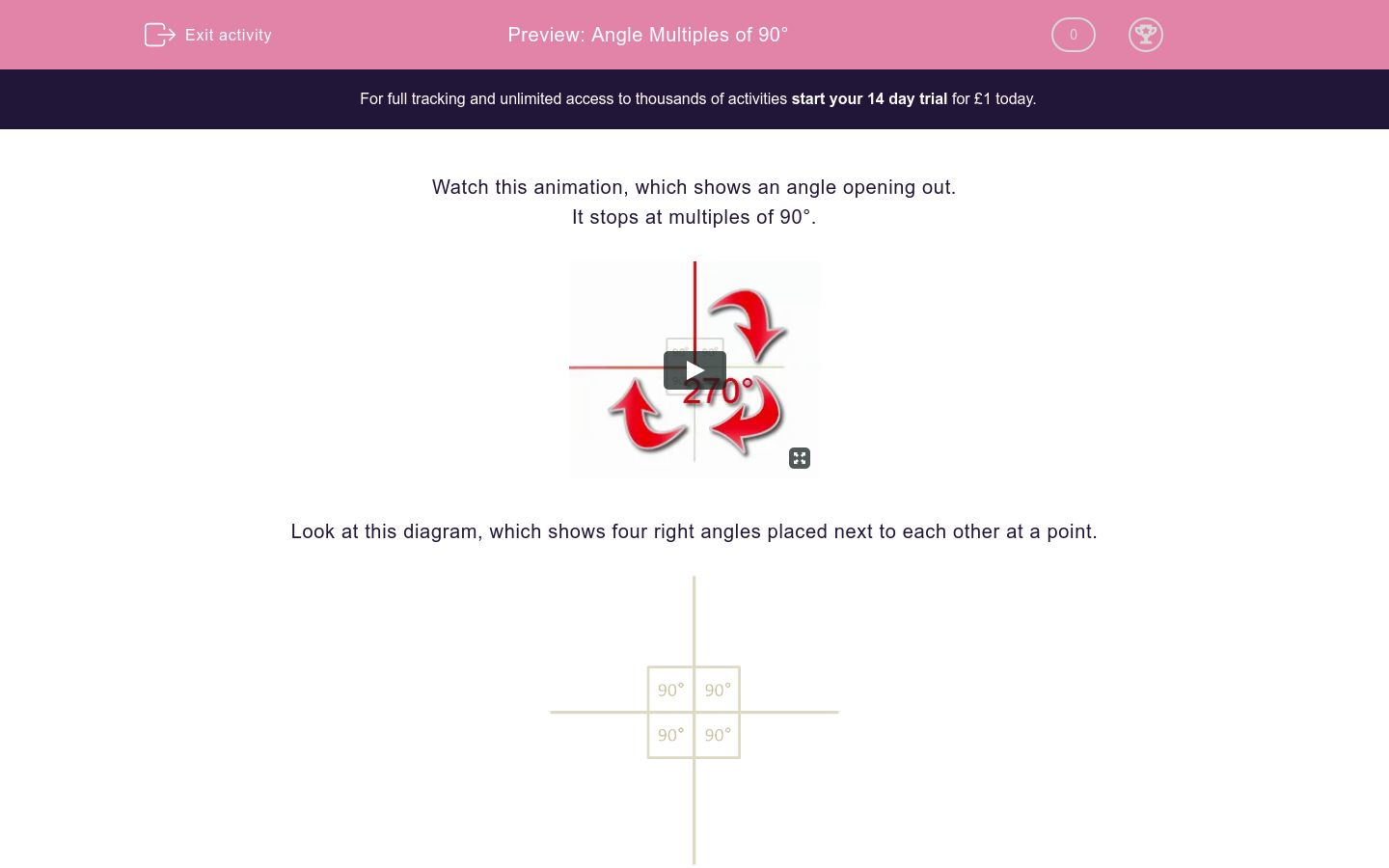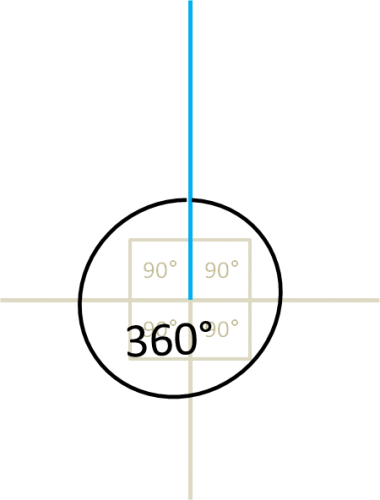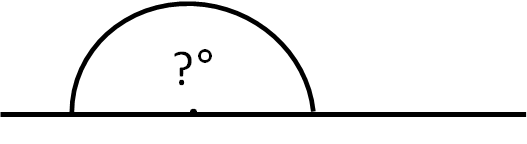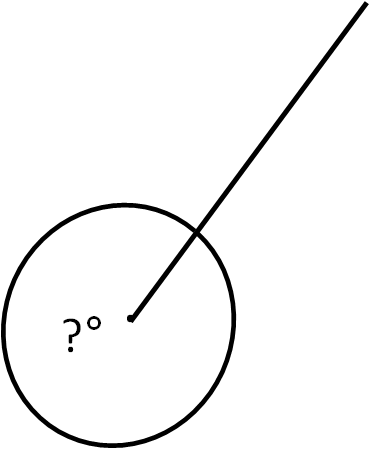# Angle Multiples of 90°

In this worksheet, students must identify angles which are multiples of 90° by counting right angles.Key stage:  KS 2

Curriculum topic:   Maths and Numerical Reasoning

Curriculum subtopic:   2D Shapes: Circles, Angles and Bearings

Difficulty level:### QUESTION 1 of 10

Watch this animation, which shows an angle opening out.

It stops at multiples of 90°.

Look at this diagram, which shows four right angles placed next to each other at a point.Here is 1 right angle, which is 90°.Here are 2 right angles put together, giving an angle of 180°.

2 × 90° = 180°Here are 3 right angles put together, giving an angle of 270°.

3 × 90° = 270°Here are 4 right angles put together, giving an angle of 360°.

4 × 90° = 360°Look at the diagram.

Select the correct value for the angle shown by the question mark.90°

180°

270°

360°

Look at the diagram.

Select the correct value for the angle shown by the question mark.90°

180°

270°

360°

Look at the diagram.

Select the correct value for the angle shown by the question mark.90°

180°

270°

360°

Look at the diagram.

Select the correct value for the angle shown by the question mark.90°

180°

270°

360°

Look at the diagram.

Select the correct value for the angle shown by the question mark.90°

180°

270°

360°

Look at the diagram.

Select the correct value for the angle shown by the question mark.90°

180°

270°

360°

Look at the diagram.

Select the correct value for the angle shown by the question mark.90°

180°

270°

360°

Look at the diagram.

Select the correct value for the angle shown by the question mark.

The angle goes right round as shown.90°

180°

270°

360°

Look at the diagram.

Select the correct value for the angle shown by the question mark.90°

180°

270°

360°

Look at the diagram.

Select the correct value for the angle shown by the question mark.90°

180°

270°

360°

• Question 1

Look at the diagram.

Select the correct value for the angle shown by the question mark.90°
EDDIE SAYS
One right angle.
• Question 2

Look at the diagram.

Select the correct value for the angle shown by the question mark.180°
EDDIE SAYS
Two right angles.
• Question 3

Look at the diagram.

Select the correct value for the angle shown by the question mark.360°
EDDIE SAYS
Four right angles.
• Question 4

Look at the diagram.

Select the correct value for the angle shown by the question mark.180°
EDDIE SAYS
Two right angles.
• Question 5

Look at the diagram.

Select the correct value for the angle shown by the question mark.270°
EDDIE SAYS
Three right angles.
• Question 6

Look at the diagram.

Select the correct value for the angle shown by the question mark.90°
EDDIE SAYS
One right angle.
• Question 7

Look at the diagram.

Select the correct value for the angle shown by the question mark.360°
EDDIE SAYS
Four right angles.
• Question 8

Look at the diagram.

Select the correct value for the angle shown by the question mark.

The angle goes right round as shown.270°
EDDIE SAYS
Three right angles.
• Question 9

Look at the diagram.

Select the correct value for the angle shown by the question mark.180°
EDDIE SAYS
Two right angles.
• Question 10

Look at the diagram.

Select the correct value for the angle shown by the question mark.270°
EDDIE SAYS
Three right angles.
---- OR ----